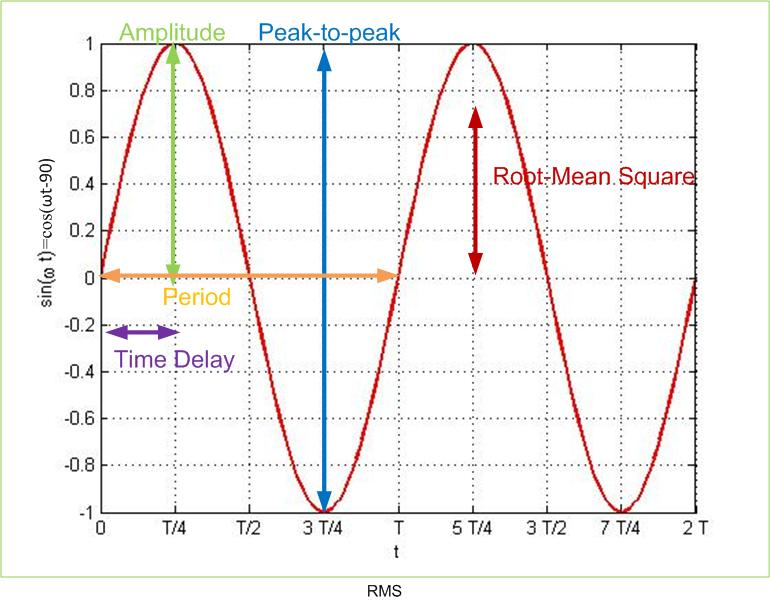$\newenvironment {prompt}{}{} \newcommand {\ungraded }{} \newcommand {\todo }{} \newcommand {\oiint }{{\large \bigcirc }\kern -1.56em\iint } \newcommand {\mooculus }{\textsf {\textbf {MOOC}\textnormal {\textsf {ULUS}}}} \newcommand {\npnoround }{\nprounddigits {-1}} \newcommand {\npnoroundexp }{\nproundexpdigits {-1}} \newcommand {\npunitcommand }{\ensuremath {\mathrm {#1}}} \newcommand {\RR }{\mathbb R} \newcommand {\R }{\mathbb R} \newcommand {\N }{\mathbb N} \newcommand {\Z }{\mathbb Z} \newcommand {\sagemath }{\textsf {SageMath}} \newcommand {\d }{\mathop {}\!d} \newcommand {\l }{\ell } \newcommand {\ddx }{\frac {d}{\d x}} \newcommand {\zeroOverZero }{\ensuremath {\boldsymbol {\tfrac {0}{0}}}} \newcommand {\inftyOverInfty }{\ensuremath {\boldsymbol {\tfrac {\infty }{\infty }}}} \newcommand {\zeroOverInfty }{\ensuremath {\boldsymbol {\tfrac {0}{\infty }}}} \newcommand {\zeroTimesInfty }{\ensuremath {\small \boldsymbol {0\cdot \infty }}} \newcommand {\inftyMinusInfty }{\ensuremath {\small \boldsymbol {\infty -\infty }}} \newcommand {\oneToInfty }{\ensuremath {\boldsymbol {1^\infty }}} \newcommand {\zeroToZero }{\ensuremath {\boldsymbol {0^0}}} \newcommand {\inftyToZero }{\ensuremath {\boldsymbol {\infty ^0}}} \newcommand {\numOverZero }{\ensuremath {\boldsymbol {\tfrac {\#}{0}}}} \newcommand {\dfn }{\textbf } \newcommand {\unit }{\mathop {}\!\mathrm } \newcommand {\eval }{\bigg [ #1 \bigg ]} \newcommand {\seq }{\left ( #1 \right )} \newcommand {\epsilon }{\varepsilon } \newcommand {\phi }{\varphi } \newcommand {\iff }{\Leftrightarrow } \DeclareMathOperator {\arccot }{arccot} \DeclareMathOperator {\arcsec }{arcsec} \DeclareMathOperator {\arccsc }{arccsc} \DeclareMathOperator {\si }{Si} \DeclareMathOperator {\scal }{scal} \DeclareMathOperator {\sign }{sign} \newcommand {\arrowvec }{{\overset {\rightharpoonup }{#1}}} \newcommand {\vec }{{\overset {\boldsymbol {\rightharpoonup }}{\mathbf {#1}}}\hspace {0in}} \newcommand {\point }{\left (#1\right )} \newcommand {\pt }{\mathbf {#1}} \newcommand {\Lim }{\lim _{\point {#1} \to \point {#2}}} \DeclareMathOperator {\proj }{\mathbf {proj}} \newcommand {\veci }{{\boldsymbol {\hat {\imath }}}} \newcommand {\vecj }{{\boldsymbol {\hat {\jmath }}}} \newcommand {\veck }{{\boldsymbol {\hat {k}}}} \newcommand {\vecl }{\vec {\boldsymbol {\l }}} \newcommand {\uvec }{\mathbf {\hat {#1}}} \newcommand {\utan }{\mathbf {\hat {t}}} \newcommand {\unormal }{\mathbf {\hat {n}}} \newcommand {\ubinormal }{\mathbf {\hat {b}}} \newcommand {\dotp }{\bullet } \newcommand {\cross }{\boldsymbol \times } \newcommand {\grad }{\boldsymbol \nabla } \newcommand {\divergence }{\grad \dotp } \newcommand {\curl }{\grad \cross } \newcommand {\lto }{\mathop {\longrightarrow \,}\limits } \newcommand {\bar }{\overline } \newcommand {\surfaceColor }{violet} \newcommand {\surfaceColorTwo }{redyellow} \newcommand {\sliceColor }{greenyellow} \newcommand {\vector }{\left \langle #1\right \rangle } \newcommand {\sectionOutcomes }{} \newcommand {\HyperFirstAtBeginDocument }{\AtBeginDocument }$

Review of Sinusoidal Signals

### Sinusoidal signal parameters

Sinusoidal signals are essential in electrical engineering as we use them to analyze and test circuit performance. All periodic signals can be represented with sinusoidal signals of different amplitudes and phases using the Fourier series.

A typical sinusoidal signal is shown in Figure sinusoid. On the y-axis is the instantaneous value of the sinusoidal voltage, and on the x-axis is time. Instantaneous values of voltage change from -1V to 1V with time. We use the following parameters to characterize sinusoidal signals: peak amplitude, peak-to-peak, average, RMS, period, time-delay, and phase. We read peak amplitude, peak-to-peak, average, and RMS values, on the y-axis in Figure sinusoid, whereas period, time delay, and phase on the x-axis.Figure 1: Vocabulary used in describing sinusoidal signals.

#### Parameters that are read on the x-axis.Figure 5: Sinusoidal signal as a function of angle $\omega t$ with a phase shift of $-\pi /4$

Calculate the time-delay in nanoseconds that you would observe on an oscilloscope if the frequency of the signal is f=0.159 GHz and the phase shift of the signal is $\theta =10^o$.
$\frac {\theta }{2*\pi *f} = \answer {10}$ ns